# 10.) Specify the electron-domain geometry for the following two species using the VSEPR model: Xe...

10.) Specify the electron-domain geometry for the following two species using the VSEPR model: XeCl4 and IO4. For each species, predict the bond angles based on the electron-domain geometry.

(a)    XeCl4
geometry:

linear

trigonal planar

bent

tetrahedral

trigonal pyramidal

trigonal bipyramidal

seesaw

T-shaped

octahedral

square pyramidal

square planar

pentagonal bipyramidal

pentagonal pyramidal

pentagonal planar

bond angle:

60°

72°

90°

109.5°

120°

180°

(b)    IO4
geometry:

linear

trigonal planar

bent

tetrahedral

trigonal pyramidal

trigonal bipyramidal

seesaw

T-shaped

octahedral

square pyramidal

square planar

pentagonal bipyramidal

pentagonal pyramidal

pentagonal planar

bond angle:

60°

72°

90°

109.5°

120°

180°

Solution:-

XeCl4

Around the xenon atom, there are 4 bonding pairs, i.e. 4 Xe−Cl bonds, and 2 lone pairs on Xe. These are arranged in the shape of an octahedron, with the non-bonding electron pairs trans with respect to each other. Because we describe molecular geometry on the basis of actual atoms, the geometry of the XeCl4 molecule is square planar.

Bond Angle of XeCl4 = 90 Degree

IO4

The geometry of IO4- is Tetrahedral.

And, The bond angle in a IO4- (Tetrahedral) is 109.5o

#### Earn Coin

Coins can be redeemed for fabulous gifts.

Similar Homework Help Questions
• ### Specify the electron-domain geometry for the following two species using the VSEPR model: KrCl4 and BrO4....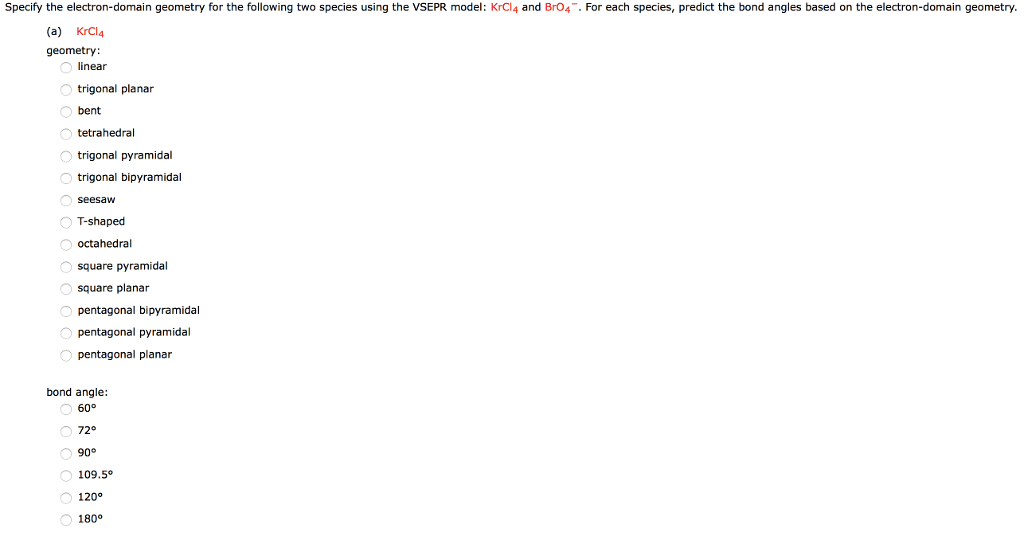Specify the electron-domain geometry for the following two species using the VSEPR model: KrCl4 and BrO4. For each species, predict the bond angles based on the electron-domain geometry. (a) KrCl4 geometry linear trigonal planar bent tetrahedral trigonal pyramidal trigonal bipyramidal T-shaped octahedral square pyramidal square planar pentagonal bipyramidal pentagonal pyramidal pentagonal planar bond angle: 72° 109.5° 120 180° (b) Br04 geometry: inear trigonal planar bent tetrahedral trigonal pyramidal trigonal bipyramidal seesaW T-shaped octahedral square pyramidal square planar pentagonal bipyramidal pentagonal...

• ### Draw the Lewis structure for AsH₃, then answer the following questions:

Draw the Lewis structure for AsH₃, then answer the following questions: A. How many valence electrons does this compound have? B. How many bonded electrons does this compound have? C. How many lone pairs of electrons does this compound have? D. How many single bonds does this compound have? E. How many double bonds does this compound have? F. What is the electron group arrangement {aka electron pair geometry}(enter one of the following: linear, trigonal planar, tetrahedral, trigonal bipyramidal, octahedral)? G. What is the molecular geometry {aka...

• ### According to VSEPR theory, XeO3 has how many regions of electron density around the central atom...

According to VSEPR theory, XeO3 has how many regions of electron density around the central atom (how many electron groups?) (enter a number from 1 to 6) ? XeO3 will have (linear, trigonal planar, tetrahedral, trigonal pyramidal, bent, trigonal bipyramidal, seesaw, T-shaped, octahedral, square pyramidal, square planar) electronic geometry, and (linear, trigonal planar, tetrahedral, trigonal pyramidal, bent, trigonal bipyramidal, seesaw, T-shaped, octahedral, square pyramidal, square planar) molecular geometry. Draw the best Lewis structure using formal charge information. How many single...

• ### Draw the Lewis structure of CIBr, showing all lone pairs. Identify the molecular geometry of CIBry...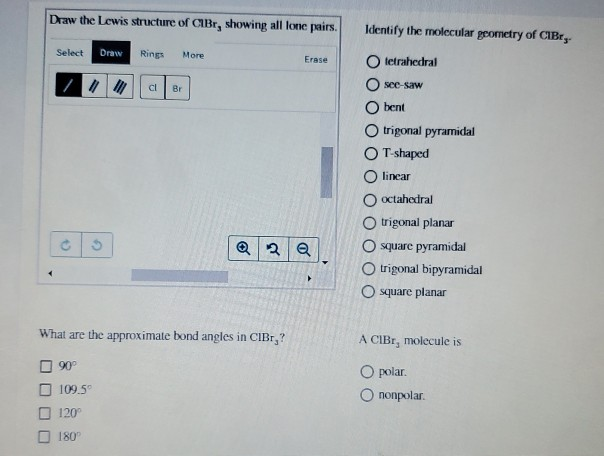Draw the Lewis structure of CIBr, showing all lone pairs. Identify the molecular geometry of CIBry Select Draw Rings More Erase tetrahedral see-saw bent trigonal pyramidal T-shaped O linear octahedral trigonal planar O square pyramidal trigonal bipyramidal square planar What are the approximate bond angles in CIBr,? A CIBr, molecule is O polar. Ononpolar. 90° 109.5 120 180

• ### olecular Geometry Saved Help Save & E Ch. Ex. 6 - Predict the Geometry Using VSEPR...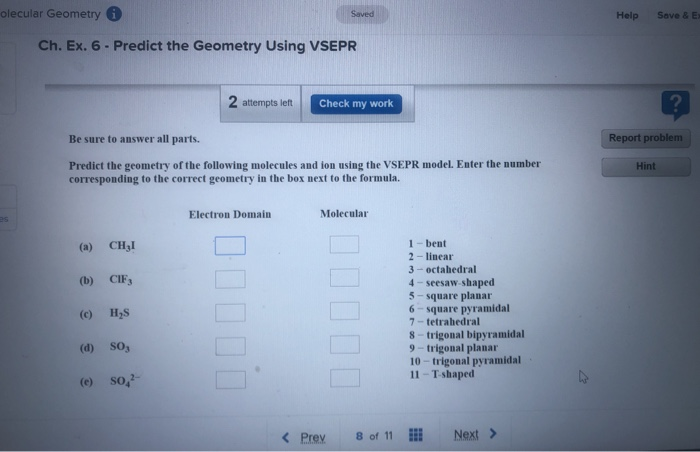olecular Geometry Saved Help Save & E Ch. Ex. 6 - Predict the Geometry Using VSEPR 2 attempts left Check my work Be sure to answer all parts. Report problem Hint Predict the geometry of the following molecules and ion using the VSEPR model. Enter the number corresponding to the correct geometry in the box next to the formula Electron Domain Molecular CHI 1 - bent 2 - linear 3-octahedral 4 - seesaw-shaped 5-square planar 6 - square pyramidal 7-tetrahedral...

• ### Draw the Lewis structure of XeCl. Include all lone pairs. Identify the molecular geometry of Xeci,...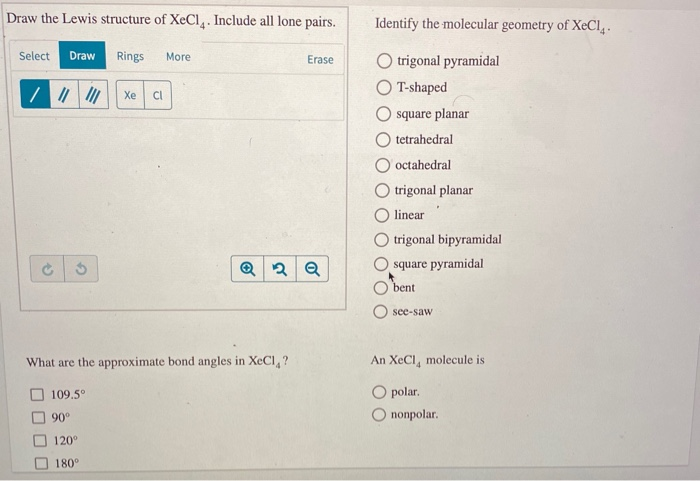Draw the Lewis structure of XeCl. Include all lone pairs. Identify the molecular geometry of Xeci, Select Draw Rings More Erase trigonal pyramidal T-shaped square planar O tetrahedral O octahedral trigonal planar linear trigonal bipyramidal square pyramidal bent O see-saw What are the approximate bond angles in XeCl,? An XeCl, molecule is O polar O nonpolar. 109.5° 90° 120° 180°

• ### Provide the correct molecular geometry for (c), given the number of lone pairs and bonding groups...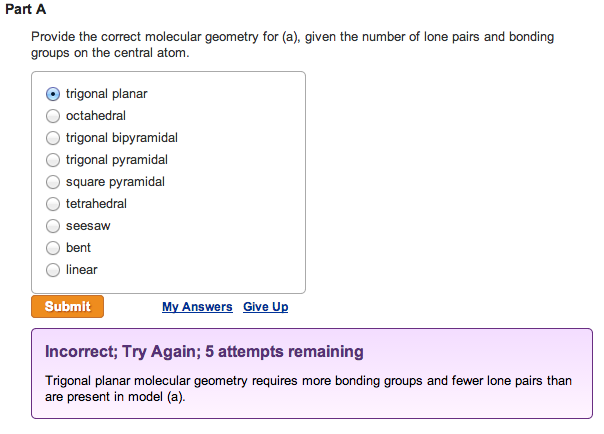Provide the correct molecular geometry for (a), given the number of lone pairs and bonding groups on the central atom. trigonal planar  octahedral  trigonal bipyramidal  trigonal pyramidal  square pyramidal  tetrahedral  seesaw  bent  linearProvide the correct molecular geometry for (b), given the number of lone pairs and bonding groups on the central atom.  trigonal bipyramidal  square pyramidal  bent  tetrahedral  trigonal planar  seesaw  trigonal pyramidal  octahedral  linear Provide the correct molecular geometry for (c), given the number of lone pairs and bonding groups on the...

• ### Please answer correctly I'll give best rating if you do correct one Electron Geomctry Name Choices:...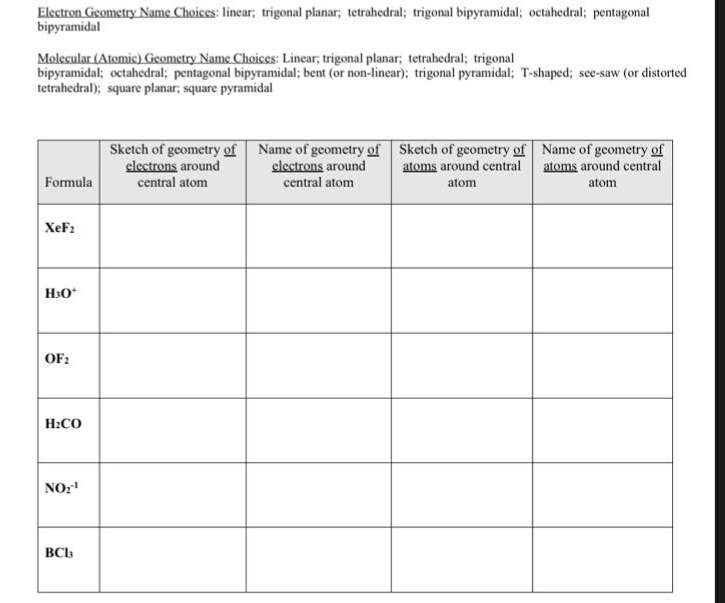Please answer correctly I'll give best rating if you do correct one Electron Geomctry Name Choices: linear; trigonal planar; tetrahedral; trigonal bipyramidal; octahedral; pentagonal bipyramidal Molecular (Atomic) Geometry Name Choices: Linear, trigonal planar; tetrahedral; trigonal bipyramidal; octahedral; pentagonal bipyramidal; bent (or non-linear); trigonal pyramidal: T-shaped; see-saw (or distorted tetrahedral); square planar, square pyramidal Sketch of geometry of Name of geometry of Sketch of geometry of Name of geometry of clectrons around central atom clectrons around atoms around central atoms around...

• ### VSEPR theory predicts which shape for the molecular structure of the following molecules or molecular ions?...

VSEPR theory predicts which shape for the molecular structure of the following molecules or molecular ions? (i.e. the arrangement of only the bonded atoms about the central atom) bent (or angular) linear octahedral see-saw square planar square pyramidal tetrahedral trigonal bipyramidal trigonal planar trigonal pyramidal T-shaped  ICl3 molecule    (The central atom is I.) bent (or angular) linear octahedral see-saw square planar square pyramidal tetrahedral trigonal bipyramidal trigonal planar trigonal pyramidal T-shaped  IO4- ion    (The central atom is I.) bent (or angular) linear...

• ### Localized Electron Bond Model -- Structure and Bonding (4 parts -- 3 molecules) The XeO3F2 molecu...

Localized Electron Bond Model -- Structure and Bonding (4 parts -- 3 molecules) The XeO3F2 molecule can be said to use which type of Xe hybrid orbitals for sigma-bonding? sp sp2 sp3 dsp3 d2sp3 VSEPR theory predicts which arrangement of bonds and lone pairs of electrons about the central Xe atom in XeO3F2? linear trigonal planar tetrahedral trigonal bipyramidal octahedral Is the XeO3F2 molecule polar or non-polar? polar non-polar 1pts Tries 0/5 The XeO3F2 molecule displays which bond angles (in...